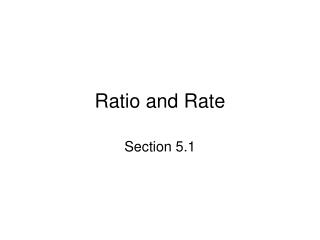DownloadDownload PresentationRatio and Rate

# Ratio and Rate

Télécharger la présentation## Ratio and Rate

- - - - - - - - - - - - - - - - - - - - - - - - - - - E N D - - - - - - - - - - - - - - - - - - - - - - - - - - -
##### Presentation Transcript

1. Ratio and Rate Section 5.1

2. Objectives • Write a fraction that shows a ratio comparison of two like measurements • Write a fraction that shows a rate comparison of two unlike measurements • Write a unit rate

3. Objective 1:Write a fraction that shows a ratio comparison of two like measurements

4. Objective 1 • Numbers can be compared by subtraction • Can be compared by division • Ratio is comparison by division • Common ways to write ratio. 30:10 30 ÷ 10 30 to 10 • First number to be compared is first number written or is the numerator. Second number second or is the denominator.

5. Objective 1 • Ratios written as fractions can be simplify, if possible • Like measurements have same unit of measure • Units are dropped in ratio with like measurements

6. Objective 2:Write a fraction that shows a rate comparison of two unlike measurements

7. Objective 2 • Fraction comparing unlike measurements is called a rate • Rate can be simplified • Units are NOT dropped

8. Objective 3: Write a unit rate

9. Objective 3 • Unit rate – rate rewritten so denominator is 1-unit measurement • To write a unit rate • Divide • Keep unlike units